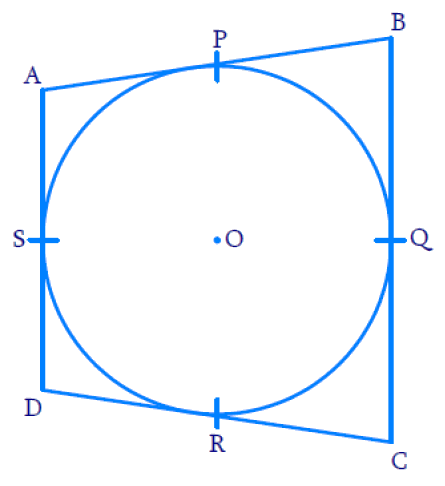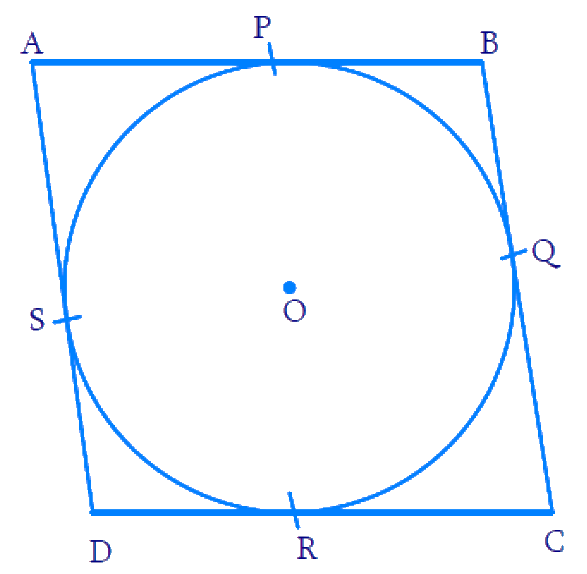# Ex.10.2 Q8 Circles Solution - NCERT Maths Class 10

Go back to  'Ex.10.2'

## Question

A quadrilateral $$ABCD$$ is drawn to circumscribe a circle (see given Figure). Prove that: $$AB + CD = AD + BC$$Video Solution
Circles
Ex 10.2 | Question 8

## Text Solution

To prove:

$$AB + CD = AD + BC$$

Reasoning:

• A tangent to a circle is a line that intersects the circle at only one point.
• Theorem 10.1: The tangent at any point of a circle is perpendicular to the radius through the point of contact.
• Theorem 10.2: The lengths of tangents drawn from an external point to a circle are equal.

Steps:We know that the length of tangents drawn from an external point of the circle are equal according to Theorem $$10.2.$$

Therefore,

$$BP = BQ$$ (Tangents from point $$B$$) $$\dots\dots\rm{(i)}$$

$$CR = CQ$$ (Tangents from point $$C$$) $$\dots \dots\rm{(ii)}$$

$$DR = DS$$  (Tangents from point $$D$$) $$\dots \dots\rm{(iii)}$$

$$AP = AS$$ (Tangents from point $$A$$$$\dots \dots\rm{(iv)}$$

Adding all the four equations, $$\text{( i) +( ii) + (iii )+ (iv)}$$ we get,

\begin{align}BP + CR + DR + AP \\= BQ + CQ + DS + AS\end{align}

On re-grouping them,

\begin{align}( A P + B P ) + ( C R + D R ) = \\( A S + D S ) + ( B Q + C Q )\, .... \,( \rm{v} )\end{align}

From the figure, we can see that:

\begin{align} {AP + BP} &= {AB} \\ {CR + DR} &= {CD} \\{AS + DS} &= {A D}\\{BQ + CQ} &= {B C}\end{align}

On substituting the above values in $$\rm{(v),}$$ we get

$$AB + CD = AD + BC$$

Hence Proved.

Learn from the best math teachers and top your exams

• Live one on one classroom and doubt clearing
• Practice worksheets in and after class for conceptual clarity
• Personalized curriculum to keep up with school